### Home > MC2 > Chapter 4 > Lesson 4.3.1 > Problem4-78

4-78.

Copy and complete each of the Diamond Problems below. The pattern used in the Diamond Problems is shown below.1.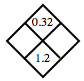What two numbers have a product of $0.32$ and a sum of $1.2$? You may have to use a guess and check method.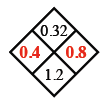1.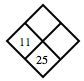$11 + ? = 25$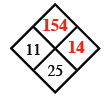1.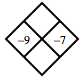What is the product and sum of $-9$ and $-7$?

1.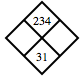See part (a).